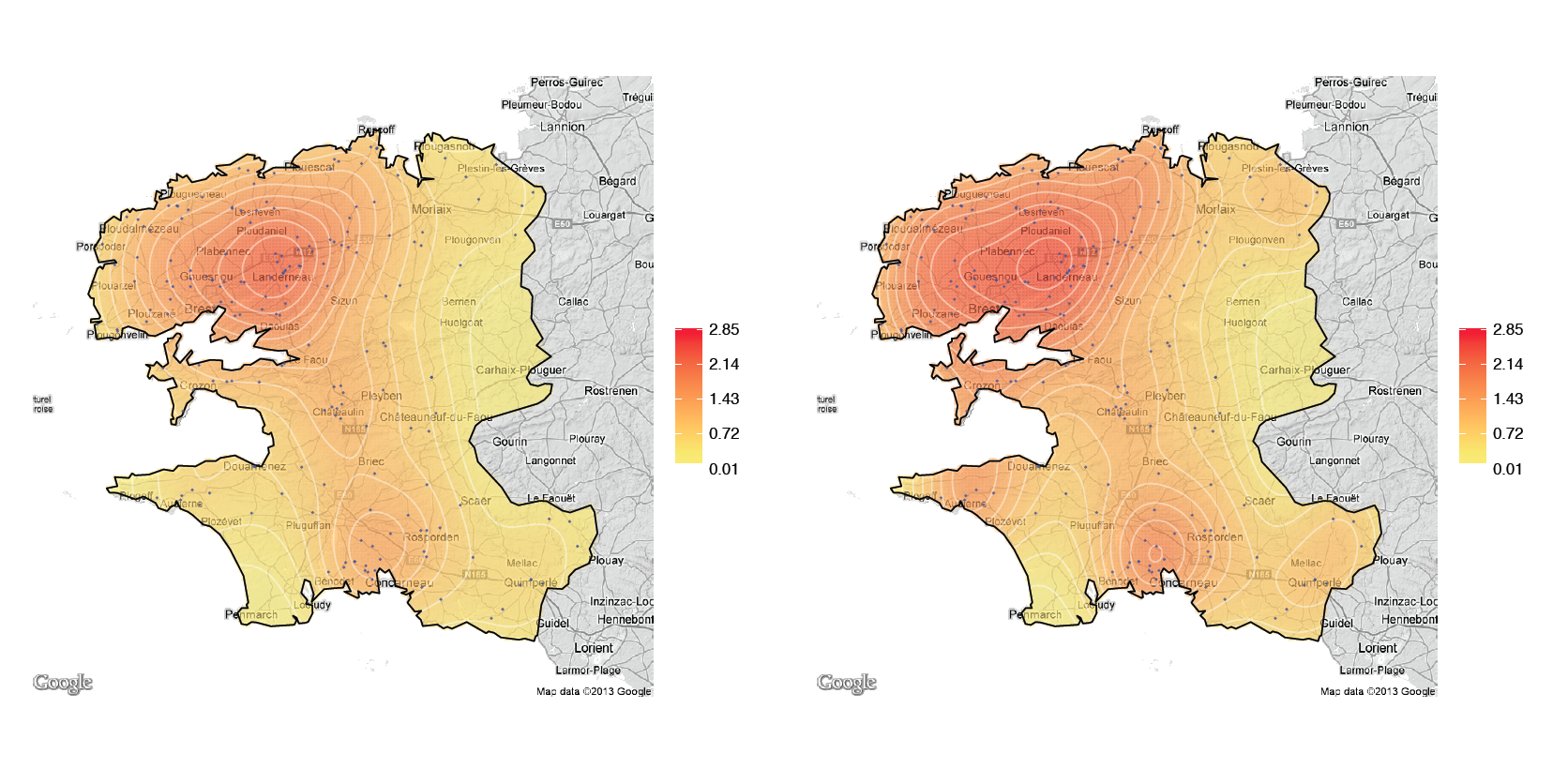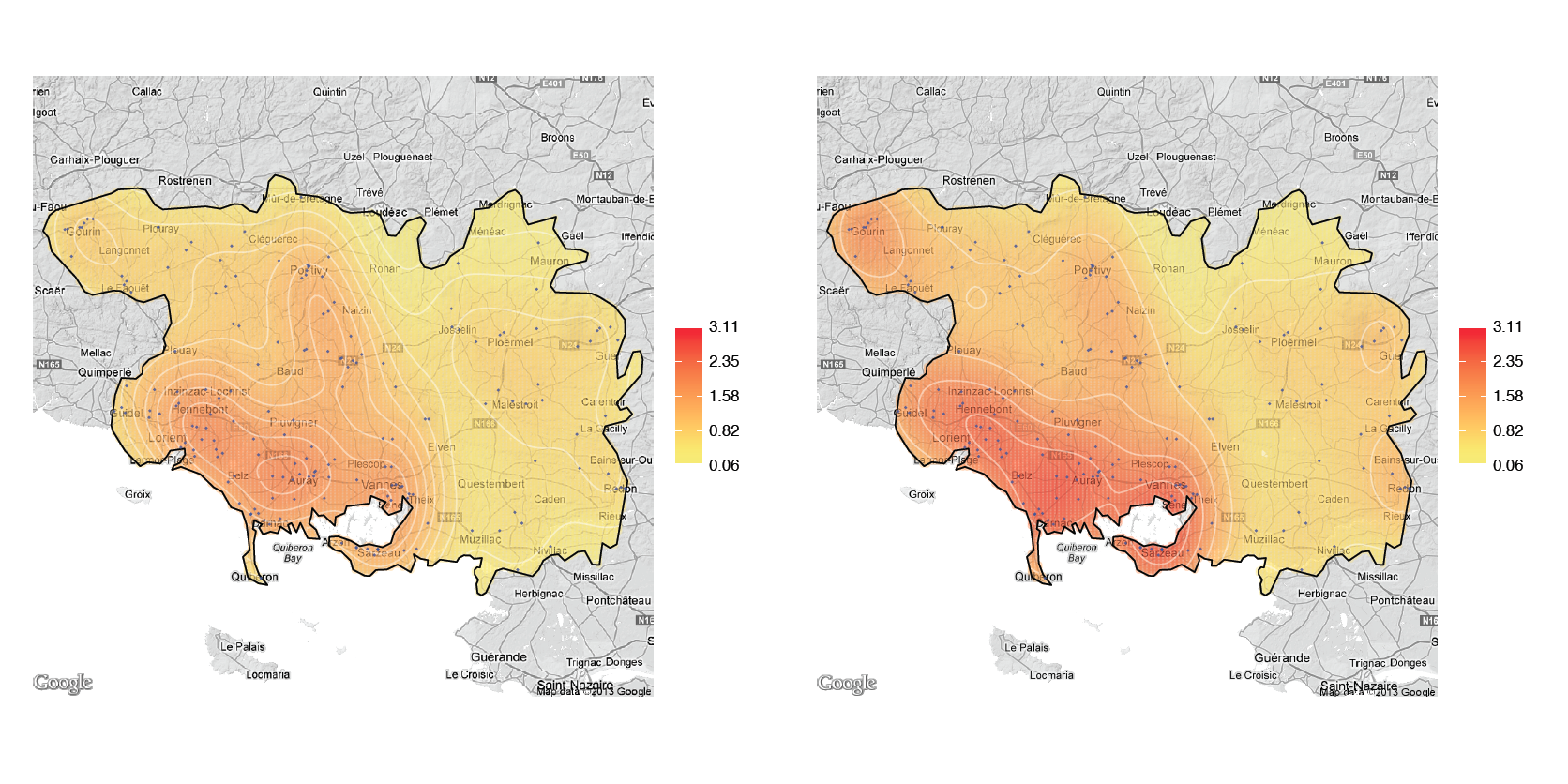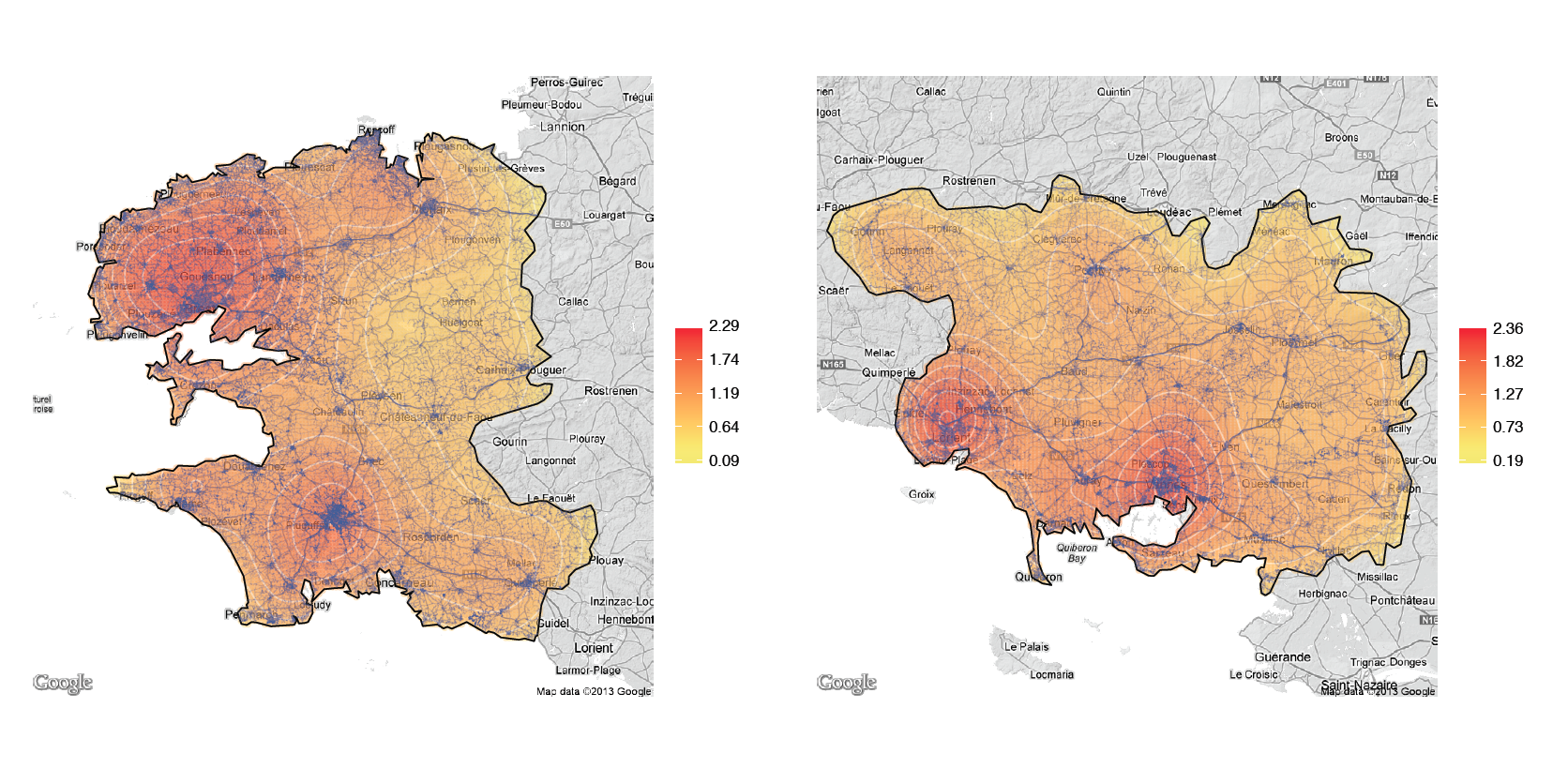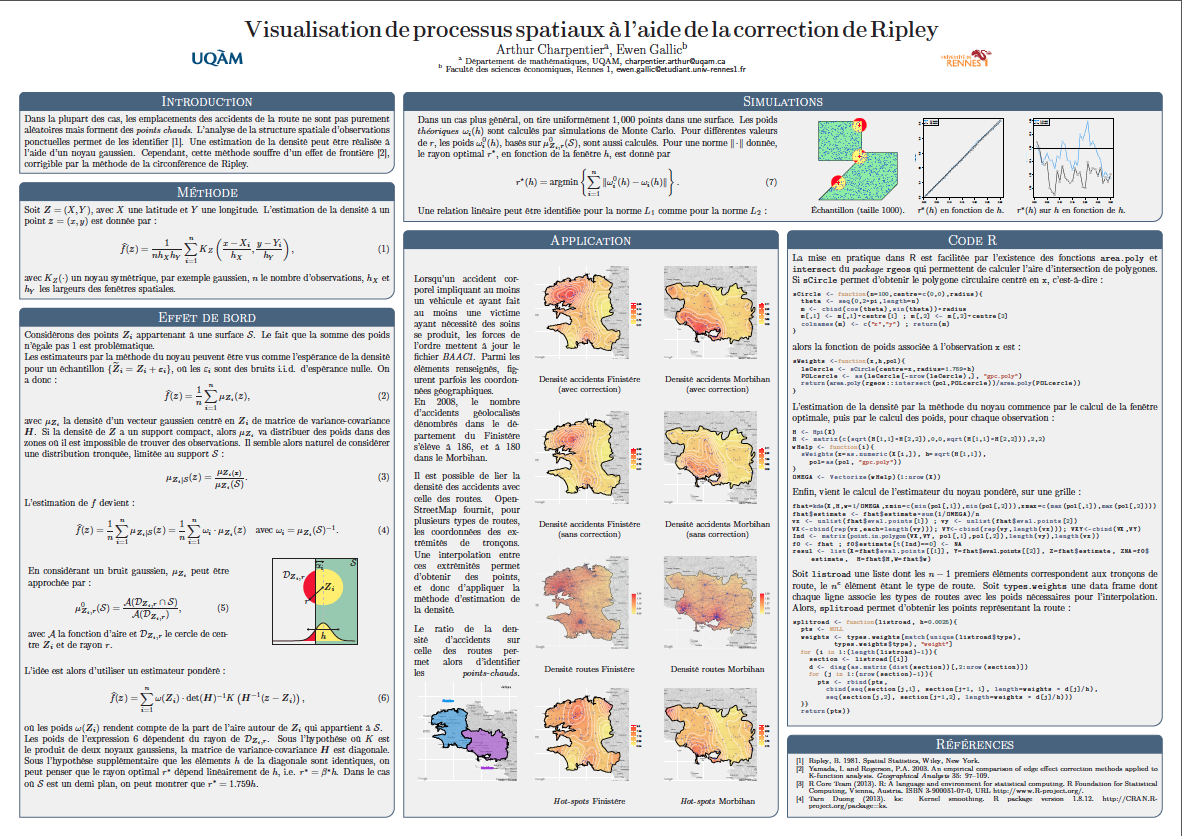In the past few weeks, Arthur Chaprentier (@freakonometrics) and I have been working again on an article untitled “Visualizing spatial processes using Ripley’s correction: an application to bodily-injury car accident location“.  The revised version of our work is available here: http://hal.archives-ouvertes.fr/hal-00725090.

Please note that this post is related to Arthur’s: http://freakonometrics.hypotheses.org/7129

We provide an easy way to correct bias of density estimation of edges of regions, by investigating and extending Ripley’s circumference method. An application to bodily-injury car accidents that happened in Finistère and Morbihan (two French regions) in 2008 is discussed in the paper, and some R codes are provided too. The purpose of this post is to explain the R code which enables to plot the results of the estimation. For those who are interested in the way to compute the estimation of the density, feel free to read the article on Hal. Please note that Arthur Charpentier wrote a nice article last year, explaining the method: http://freakonometrics.hypotheses.org/2416, but be aware that some tiny changes have been made to the functions.

There were 186 geolocated bodily-injury car accidents in 2008 in Finistère, and 180 in Morbihan. Their spatial distribution can be viewed on the maps below, with a standard kernel on the left and corrected one on the right.Now, let’s have a look at the R code, shall we? It has been tested on R 3.0.1 and R 2.15.3 too. First, you’ll need to load the following packages: ggplot2 and ggmap.

Let us assume that the result of the density estimation is stored in the object called result

Let us send a query to Google Maps API to get a nice map.

theMap <- get_map(location = c(left = min(pol[, 1]), bottom = min(pol[,
2]), right = max(pol[, 1]), top = max(pol[, 2])), source = "google",
messaging = F, color = "bw")


Since we are using a function that relies on ggplot2 grammar, we need to put the data in the right format. To do so, we’ve created this little function :

getMelt <- function(smoothed) {
res <- melt(smoothed$ZNA) res[, 1] <- smoothed$X[res[, 1]]
res[, 2] <- smoothed$Y[res[, 2]] names(res) <- list("X", "Y", "ZNA") return(res) }  Let us apply this function to the list containing the results of the estimation : smCont <- getMelt(result)  Now, we should define a color scale and some breaks. breaks <- seq(min(result$ZNA, na.rm = TRUE) * 0.95, max(result$ZNA, na.rm = TRUE) * 1.05, length = 21) col <- rev(heat.colors(20))  We should also change the labels we want to display in the legend : theLabels <- round(breaks, 2) indLabels <- floor(seq(1, length(theLabels), length.out = 5)) indLabels[length(indLabels)] <- length(theLabels) theLabels <- as.character(theLabels[indLabels]) theLabels[theLabels == "0"] <- " 0.00 "  Finally, the map can be built : P <- ggmap(theMap) P <- P + geom_point(aes(x = X, y = Y, col = ZNA), alpha = 0.3, data = smCont[!is.na(smCont$ZNA), ], na.rm = TRUE)


If one wants to add a contour :

P <- P + geom_contour(data = smCont[!is.na(smCont$ZNA), ], aes(x = X, y = Y, z = ZNA), alpha = 0.5, colour = " white ")  If you display the map now, there is something missing: colors! So, the plot has to be updated. P <- P + scale_colour_gradient(name = "", low = " yellow ", high = "red", breaks = breaks[indLabels], limits = range(breaks), labels = theLabels)  We might want to remove some elements, such as the axis ticks and legends: P <- P + theme(panel.grid.minor = element_line(colour = NA), panel.grid.minor = element_line(colour = NA), panel.background = element_rect(fill = NA, colour = NA), axis.text.x = element_blank(), axis.text.y = element_blank(), axis.ticks.x = element_blank(), axis.ticks.y = element_blank(), axis.title = element_blank(), rect = element_blank())  And, to finish, let us draw the border of the polygon (assuming the polygon is named pol): polDF <- data.frame(pol) colnames(polDF) <- list("lon", "lat") (P <- P + geom_polygon(data = polDF, mapping = (aes(x = lon, y = lat)), colour = " black ", fill = NA))  To account for traffic, we’ve downloaded road sections from http://www.geofabrik.de/index.html, which provides files based on OpenStreetMap data. Each observation is a section of a road, and contains a few points identified by their geographical coordinates that allow to draw lines. We interpolate points between the extremities of road sections, weighting the distance separating each points according to the importance of the road. Hence, these points are used as a proxy for traffic. splitroad <- function(listroad, h = 0.0025) { pts = NULL weights <- types.weights[match(unique(listroad$type), types.weights\$type), "weight"]
for (i in 1:(length(listroad) - 1)) {
for (j in 1:(nrow(listroad[[i]]) - 1)) {
pts = rbind(pts, cbind(seq((listroad[[i]])[j, 1], (listroad[[i]])[j + 1, 1], length = weights * d[j]/h),
}
}
return(pts)
}


The result can be seen on the maps below.That’s pretty much it. Oh, by the way, I will be presenting a poster on which Arthur and I have been working during the 2nd French R Meeting in Lyon, on the 27th-28th of June 2013. f you want to have a look, click on the image down below to view a PDF version (the document is written in French).